# 1 Introdution

DALEX is designed to work with various black-box models like tree ensembles, linear models, neural networks etc. Unfortunately R packages that create such models are very inconsistent. Different tools use different interfaces to train, validate and use models.

In this vignette we will show explanations for models from caret package (Jed Wing et al. 2016).

# 2 Regression use case - apartments data

``````library(DALEX)
library(caret)``````

To illustrate applications of DALEX to regression problems we will use an artificial dataset `apartments` available in the DALEX package. Our goal is to predict the price per square meter of an apartment based on selected features such as construction year, surface, floor, number of rooms, district. It should be noted that four of these variables are continuous while the fifth one is a categorical one. Prices are given in Euro.

``````data(apartments)
``````##   m2.price construction.year surface floor no.rooms    district
## 1     5897              1953      25     3        1 Srodmiescie
## 2     1818              1992     143     9        5     Bielany
## 3     3643              1937      56     1        2       Praga
## 4     3517              1995      93     7        3      Ochota
## 5     3013              1992     144     6        5     Mokotow
## 6     5795              1926      61     6        2 Srodmiescie``````

## 2.1 The explain() function

The first step of using the DALEX package is to wrap-up the black-box model with meta-data that unifies model interfacing.

Below, we use the caret function `train()` to fit 3 models: random forest, gradient boosting machine model, and neutral network.

``````set.seed(123)
regr_rf <- train(m2.price~., data = apartments, method="rf", ntree = 100)

regr_gbm <- train(m2.price~. , data = apartments, method="gbm")

regr_nn <- train(m2.price~., data = apartments,
method = "nnet",
linout = TRUE,
preProcess = c('center', 'scale'),
maxit = 500,
tuneGrid = expand.grid(size = 2, decay = 0),
trControl = trainControl(method = "none", seeds = 1))``````

To create an explainer for these models it is enough to use `explain()` function with the model, data and y parameters. Validation dataset for the models is `apartmentsTest` data from the DALEX package.

``````data(apartmentsTest)

explainer_regr_rf <- DALEX::explain(regr_rf, label="rf",
data = apartmentsTest, y = apartmentsTest\$m2.price,
colorize = FALSE)``````
``````## Preparation of a new explainer is initiated
##   -> model label       :  rf
##   -> data              :  9000  rows  6  cols
##   -> target variable   :  9000  values
##   -> data              :  A column identical to the target variable `y` has been found in the `data`.  (  WARNING  )
##   -> data              :  It is highly recommended to pass `data` without the target variable column
##   -> predict function  :  yhat.train  will be used (  default  )
##   -> predicted values  :  numerical, min =  1689.261 , mean =  3499.263 , max =  6399.074
##   -> residual function :  difference between y and yhat (  default  )
##   -> residuals         :  numerical, min =  -675.6937 , mean =  12.26021 , max =  754.6977
##   -> model_info        :  package , ver. , task Regression (  default  )
##   A new explainer has been created!``````
``````explainer_regr_gbm <- DALEX::explain(regr_gbm, label = "gbm",
data = apartmentsTest, y = apartmentsTest\$m2.price,
colorize = FALSE)``````
``````## Preparation of a new explainer is initiated
##   -> model label       :  gbm
##   -> data              :  9000  rows  6  cols
##   -> target variable   :  9000  values
##   -> data              :  A column identical to the target variable `y` has been found in the `data`.  (  WARNING  )
##   -> data              :  It is highly recommended to pass `data` without the target variable column
##   -> predict function  :  yhat.train  will be used (  default  )
##   -> predicted values  :  numerical, min =  1695.891 , mean =  3506.852 , max =  6455.727
##   -> residual function :  difference between y and yhat (  default  )
##   -> residuals         :  numerical, min =  -475.1496 , mean =  4.671189 , max =  519.1025
##   -> model_info        :  package , ver. , task Regression (  default  )
##   A new explainer has been created!``````
``````explainer_regr_nn <- DALEX::explain(regr_nn, label = "nn",
data = apartmentsTest, y = apartmentsTest\$m2.price,
colorize = FALSE)``````
``````## Preparation of a new explainer is initiated
##   -> model label       :  nn
##   -> data              :  9000  rows  6  cols
##   -> target variable   :  9000  values
##   -> data              :  A column identical to the target variable `y` has been found in the `data`.  (  WARNING  )
##   -> data              :  It is highly recommended to pass `data` without the target variable column
##   -> predict function  :  yhat.train  will be used (  default  )
##   -> predicted values  :  numerical, min =  2929.134 , mean =  3489.192 , max =  4342.255
##   -> residual function :  difference between y and yhat (  default  )
##   -> residuals         :  numerical, min =  -1326.212 , mean =  22.33205 , max =  2336.745
##   -> model_info        :  package , ver. , task Regression (  default  )
##   A new explainer has been created!``````

## 2.2 Model performance

Function `model_performance()` calculates predictions and residuals for validation dataset.

``````mp_regr_rf <- model_performance(explainer_regr_rf)
mp_regr_gbm <- model_performance(explainer_regr_gbm)
mp_regr_nn <- model_performance(explainer_regr_nn)``````

Generic function `print()` returns quantiles for residuals.

``mp_regr_rf``
``````##         0%        10%        20%        30%        40%        50%
## -754.69767 -209.95295  -81.12960  -34.76663  -10.41347    7.54125
##        60%        70%        80%        90%       100%
##   25.68403   49.04287   80.66407  138.01935  675.69367``````

Generic function `plot()` shows reversed empirical cumulative distribution function for absolute values from residuals. Plots can be generated for one or more models.

``plot(mp_regr_rf, mp_regr_nn, mp_regr_gbm)``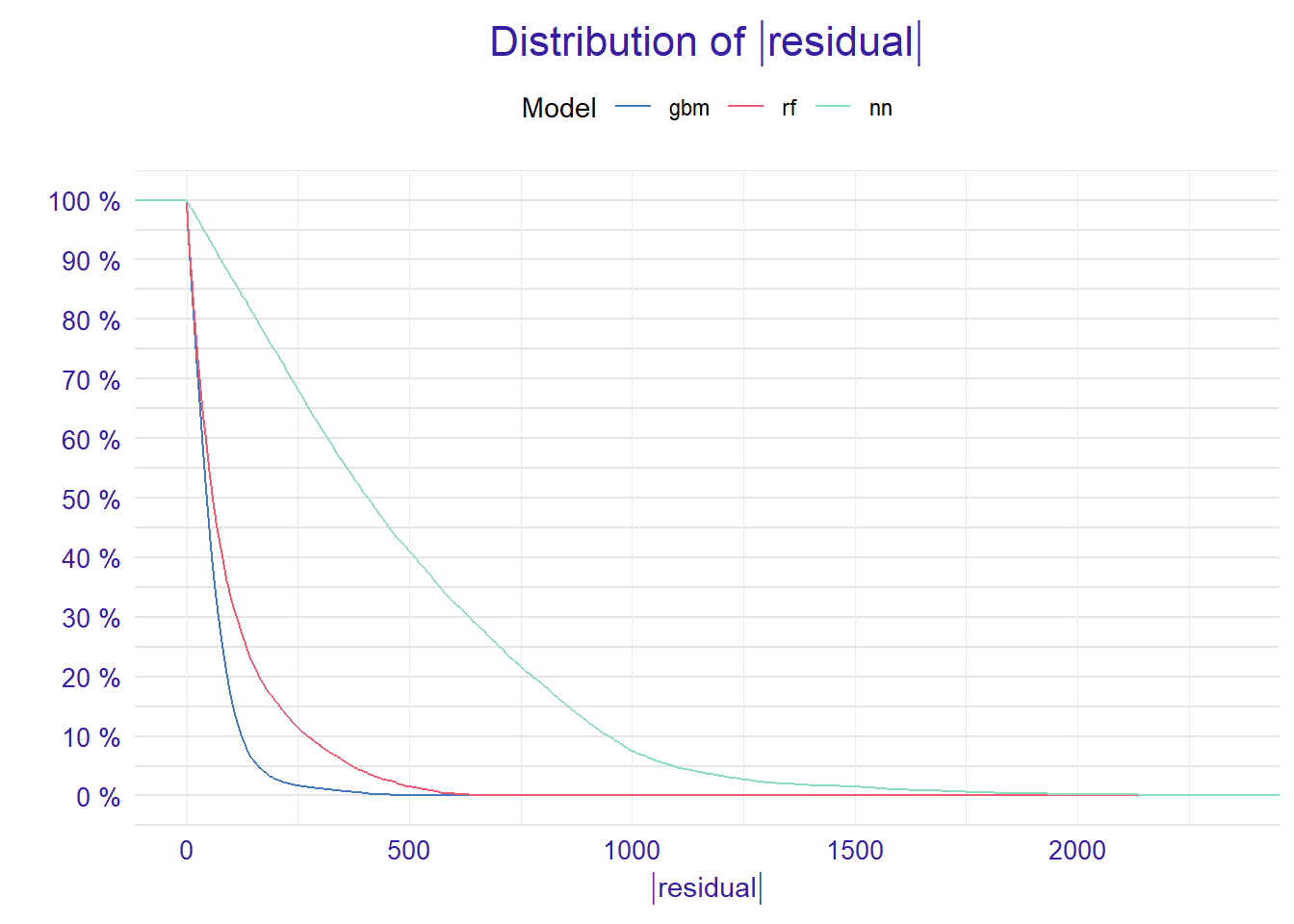The figure above shows that majority of residuals for random forest and gbm are smaller than residuals for the neural network.

We are also able to use the `plot()` function to get an alternative comparison of residuals. Setting the `geom = "boxplot"` parameter we can compare the distribution of residuals for selected models.

``plot(mp_regr_rf, mp_regr_nn, mp_regr_gbm, geom = "boxplot")``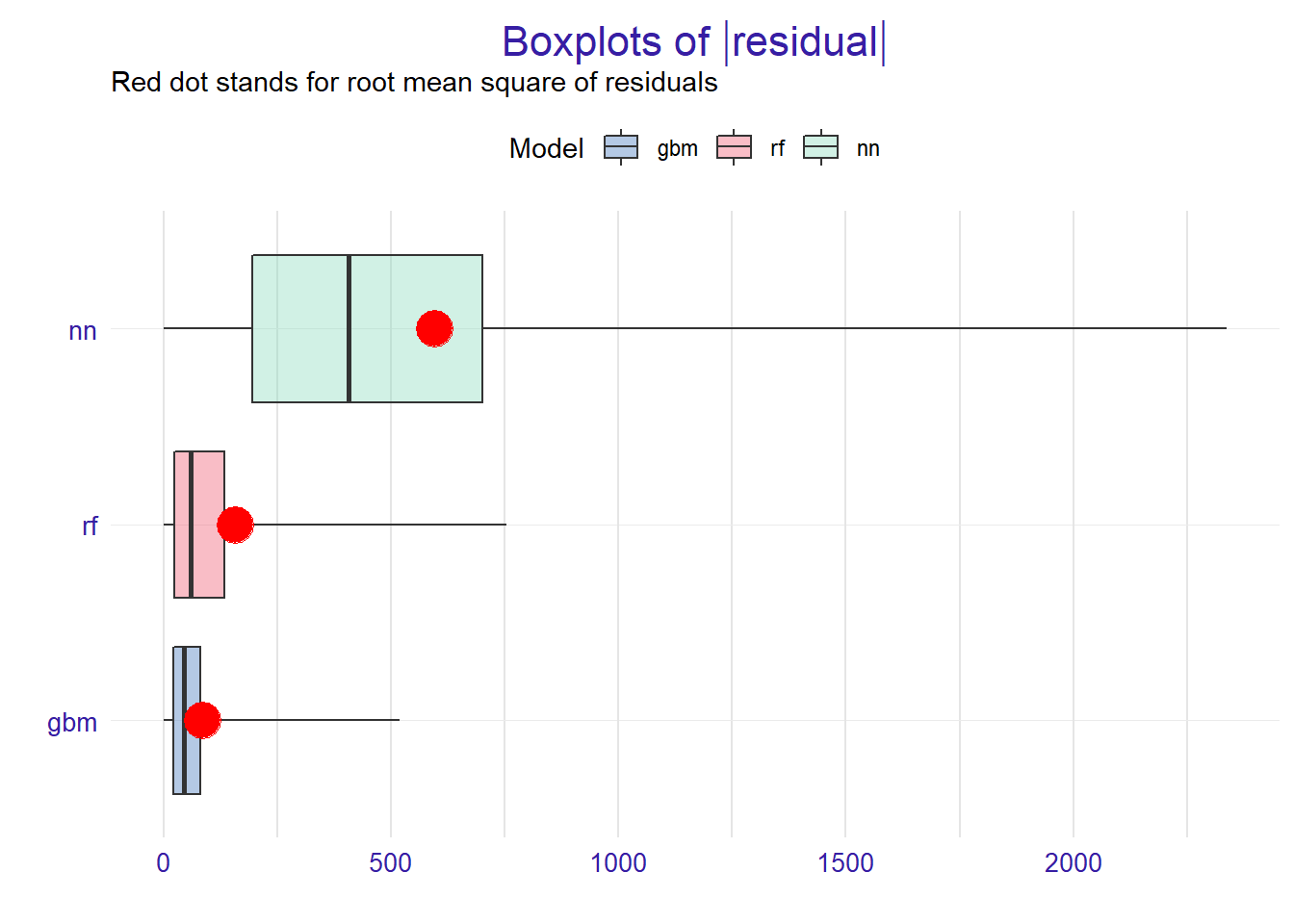## 2.3 Variable importance

Using he DALEX package we are able to better understand which variables are important.

Model agnostic variable importance is calculated by means of permutations. We simply substract the loss function calculated for validation dataset with permuted values for a single variable from the loss function calculated for validation dataset.

This method is implemented in the `variable_importance()` function.

``````vi_regr_rf <- variable_importance(explainer_regr_rf, loss_function = loss_root_mean_square)
vi_regr_gbm <- variable_importance(explainer_regr_gbm, loss_function = loss_root_mean_square)
vi_regr_nn <- variable_importance(explainer_regr_nn, loss_function = loss_root_mean_square)``````

We can compare all models using the generic `plot()` function.

``plot(vi_regr_rf, vi_regr_gbm, vi_regr_nn)``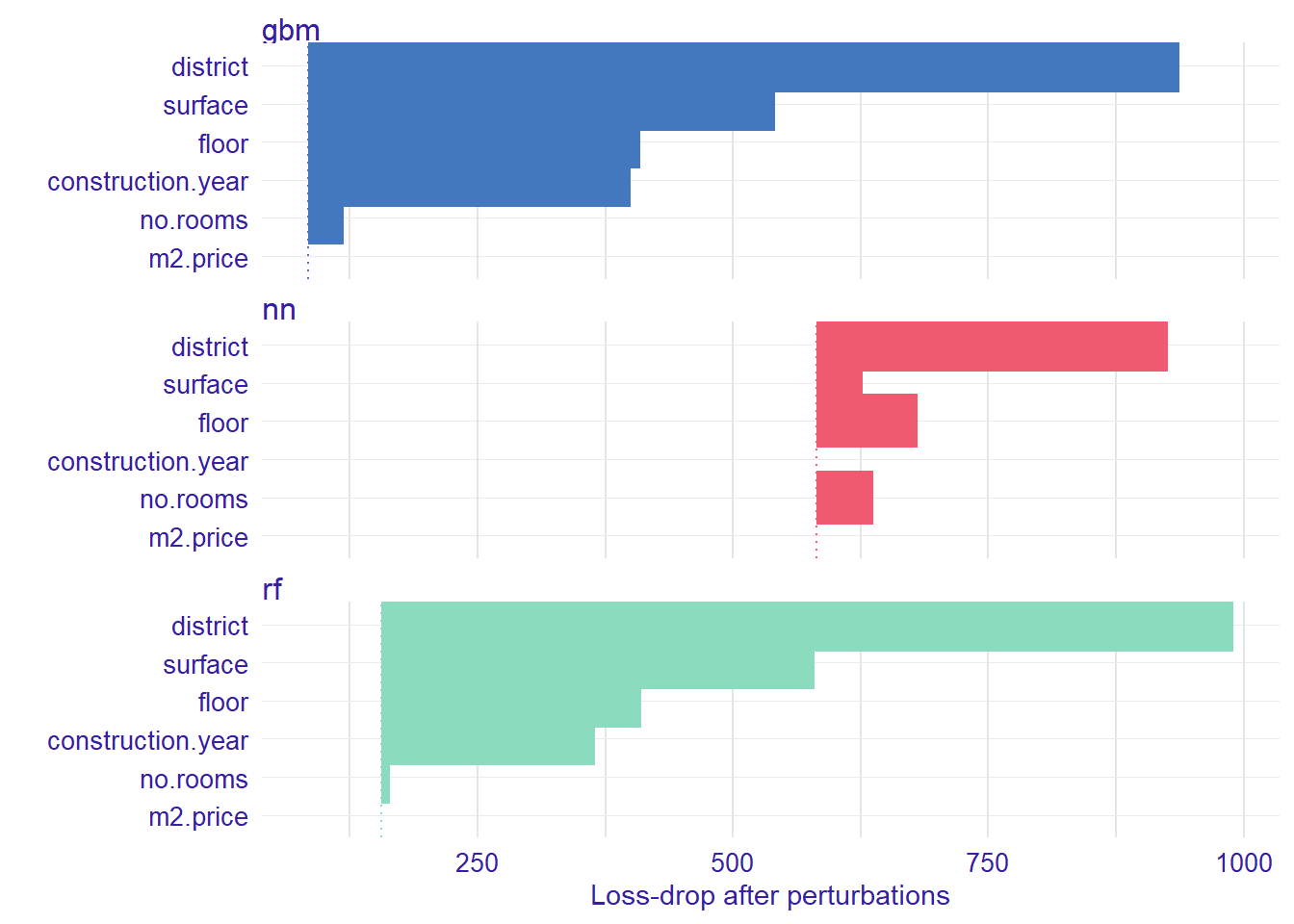Left edges of intervals start in full model, as we can see performances for random forest and gbm are similar, while neutral network has worse performace.

Length of the interval coresponds to a variable importance. Longer interval means larger loss, so the variable is more important. For random forest and gbm the rankings of the important variables are the same.

## 2.4 Variable response

Explainers presented in this section are designed to better understand the relation between a variable and model output.

For more details of methods desribed in this section see Variable response section in DALEX docs.

### 2.4.1 Partial Dependence Plot

Partial Dependence Plots (PDP) are one of the most popular methods for exploration of the relation between a continuous variable and the model outcome.

Function `variable_response()` with the parameter `type = "pdp"` calls `pdp::partial()` function to calculate PDP response.

``````pdp_regr_rf  <- variable_response(explainer_regr_rf, variable =  "construction.year", type = "pdp")
pdp_regr_gbm  <- variable_response(explainer_regr_gbm, variable =  "construction.year", type = "pdp")
pdp_regr_nn  <- variable_response(explainer_regr_nn, variable =  "construction.year", type = "pdp")

plot(pdp_regr_rf, pdp_regr_gbm, pdp_regr_nn)``````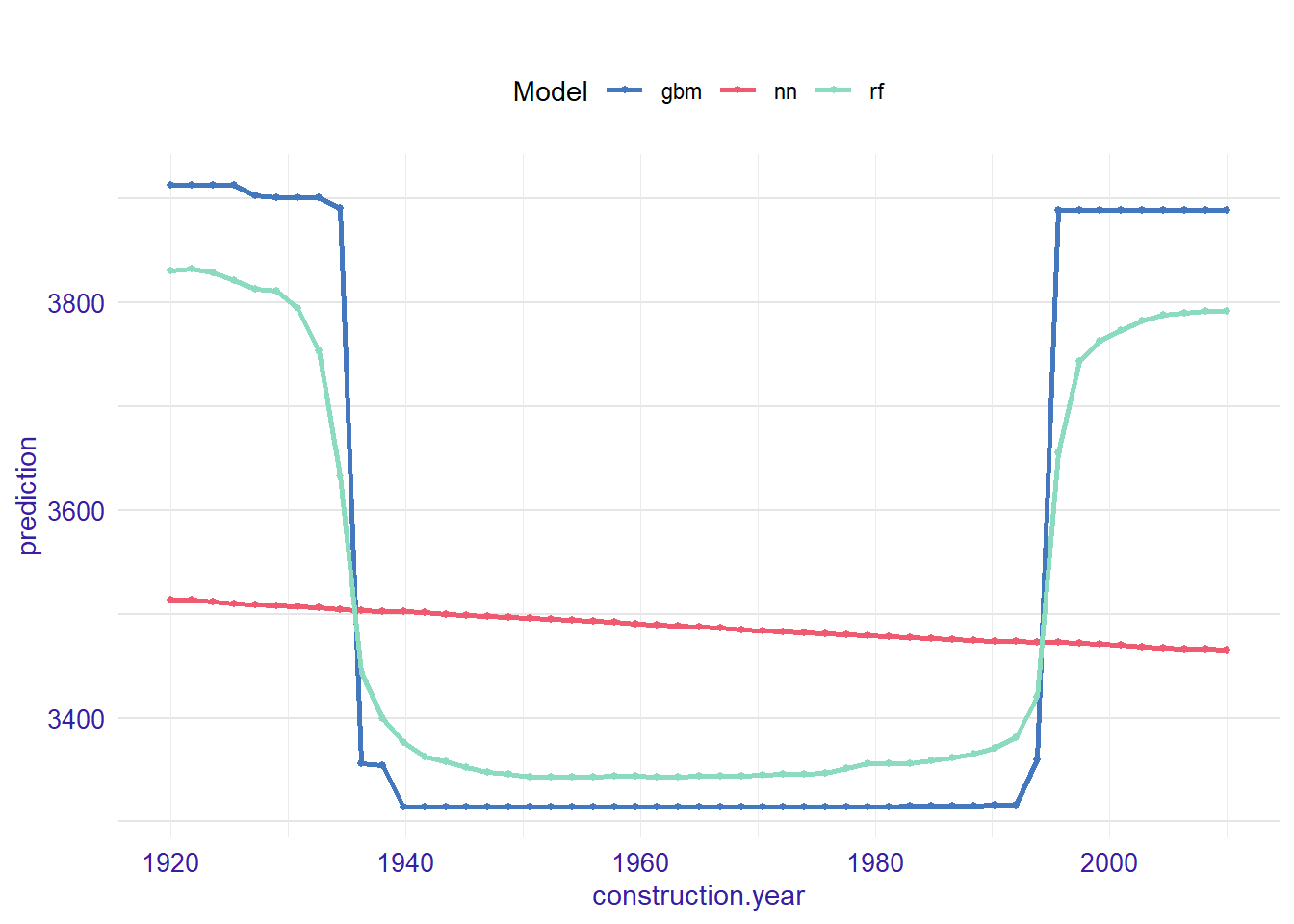We use PDP plots to compare our 3 models. As we can see above performance of random forest and gbm is very similar. It looks like they capture the non-linear relation which wasn’t captured by neutral network.

### 2.4.2 Acumulated Local Effects plot

Acumulated Local Effects (ALE) plot is the extension of PDP, that is more suited for highly correlated variables.

Function `variable_response()` with the parameter `type = "ale"` calls `ALEPlot::ALEPlot()` function to calculate the ALE curve for the variable `construction.year`.

``````ale_regr_rf  <- variable_response(explainer_regr_rf, variable =  "construction.year", type = "ale")
ale_regr_gbm  <- variable_response(explainer_regr_gbm, variable =  "construction.year", type = "ale")
ale_regr_nn  <- variable_response(explainer_regr_nn, variable =  "construction.year", type = "ale")

plot(ale_regr_rf, ale_regr_gbm, ale_regr_nn)``````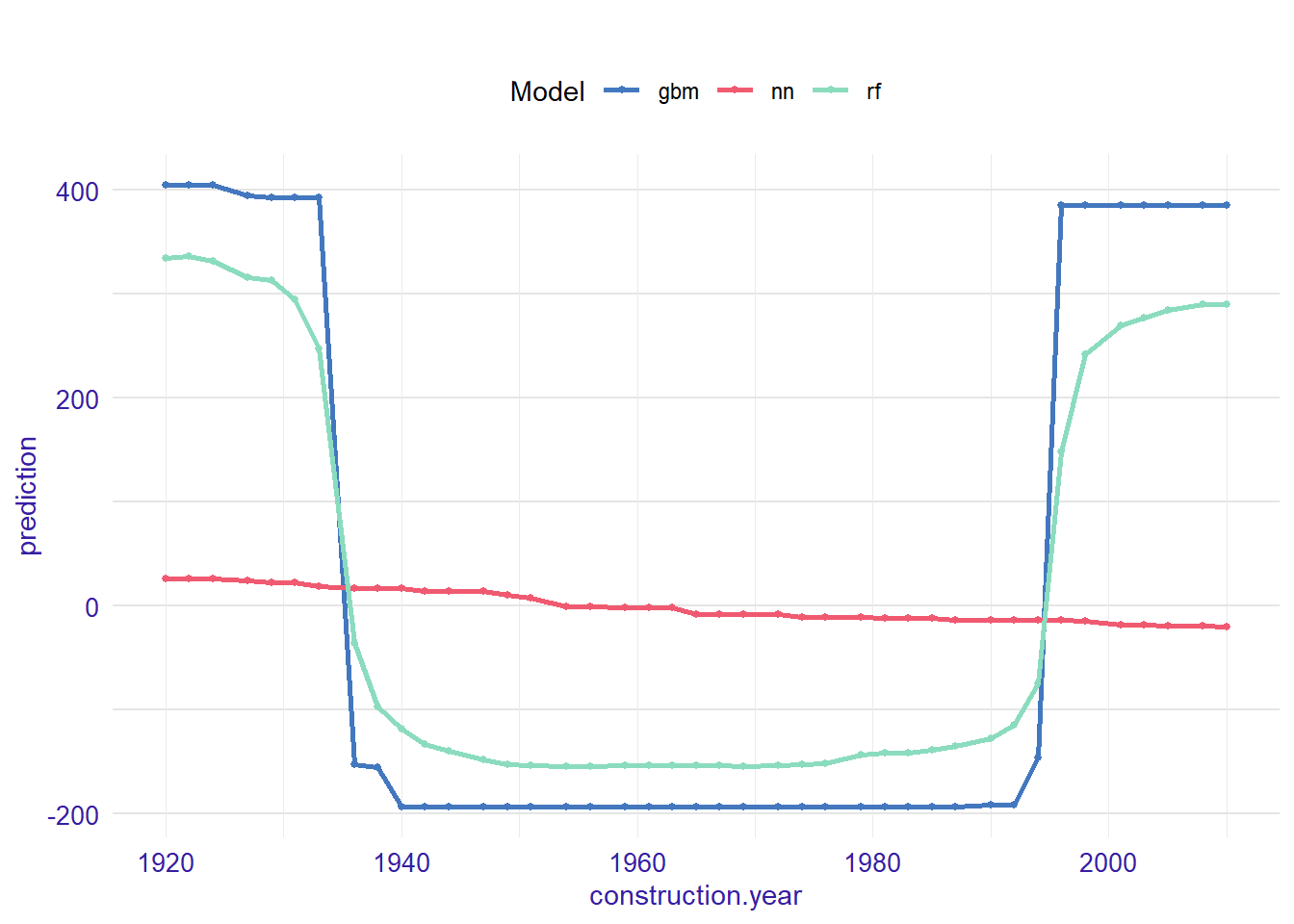### 2.4.3 Merging Path Plots

Merging Path Plot is a method for exploration of a relation between a categorical variable and model outcome.

Function `variable_response()` with the parameter `type = "factor"` calls `factorMerger::mergeFactors()` function.

``````mpp_regr_rf  <- variable_response(explainer_regr_rf, variable =  "district", type = "factor")
mpp_regr_gbm  <- variable_response(explainer_regr_gbm, variable =  "district", type = "factor")
mpp_regr_nn  <- variable_response(explainer_regr_nn, variable =  "district", type = "factor")

plot(mpp_regr_rf, mpp_regr_gbm, mpp_regr_nn)``````